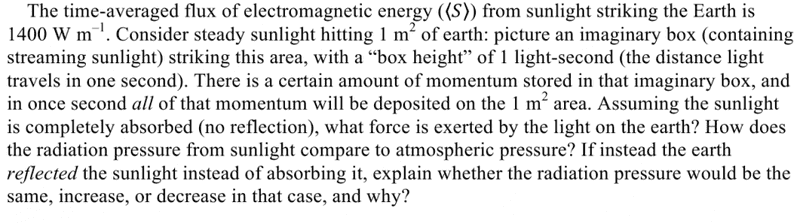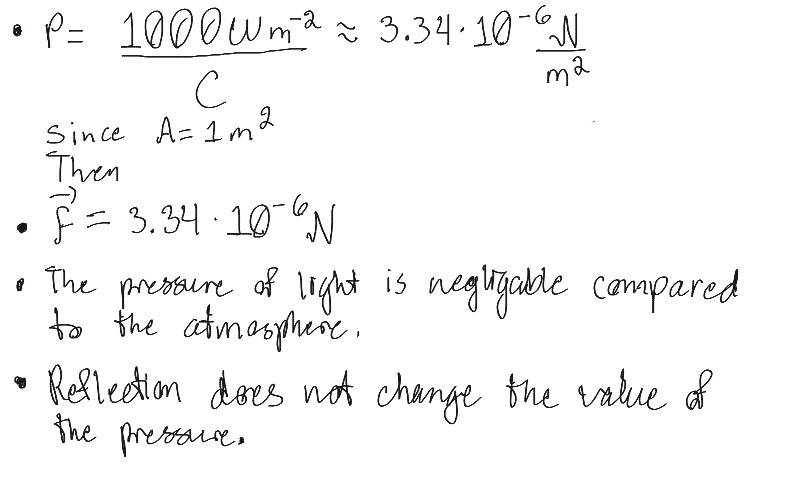# Momentum in a Box of Light

• DrPapper

## Homework Statement## Homework Equations

<S>=c$\epsilon$E2/2

$\Delta P = A \Delta t <S>/c$

## The Attempt at a Solution

My question is if we need the "box height"? This is my work which I'm assuming is wrong. :CIf there's another problem like this on here please put a link. I didn't see any, but my search terms may not have been ideal "Pressure of light in a box".

What is the momentum of the light? Why did 1400 W m-2 become 1000 W m-2? You didn't say why reflection doesn't change the answer.